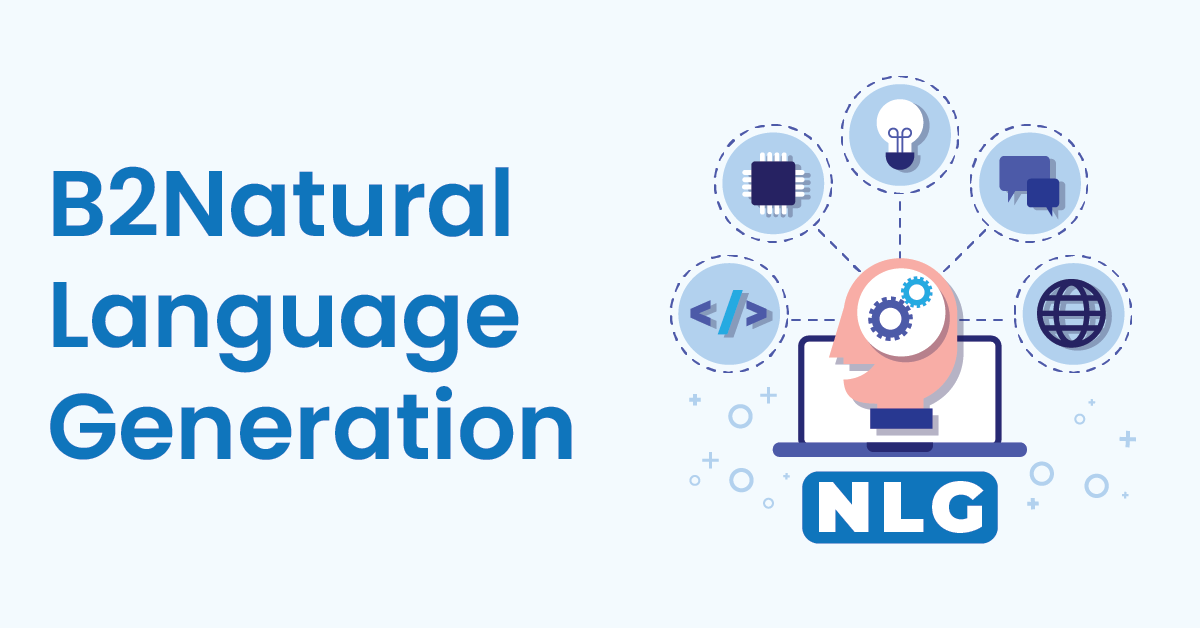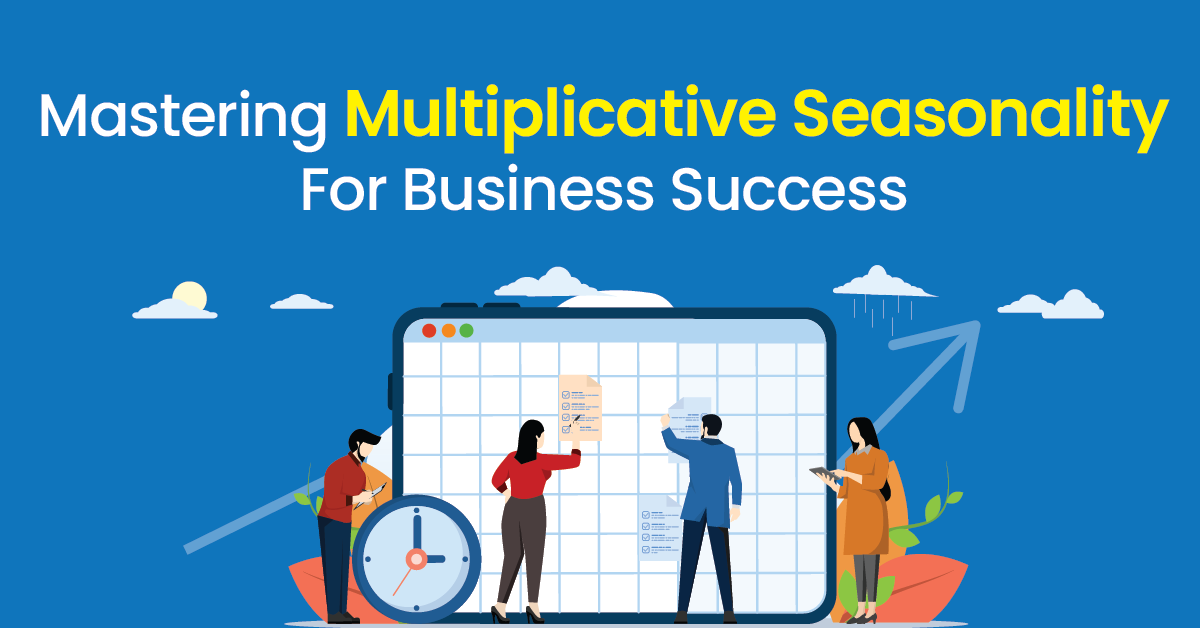Home / Blog / Data Science / Simple Linear Regression : Introduction & Applications

# Simple Linear Regression : Introduction & Applications

• October 13, 2023
• 917
• 2584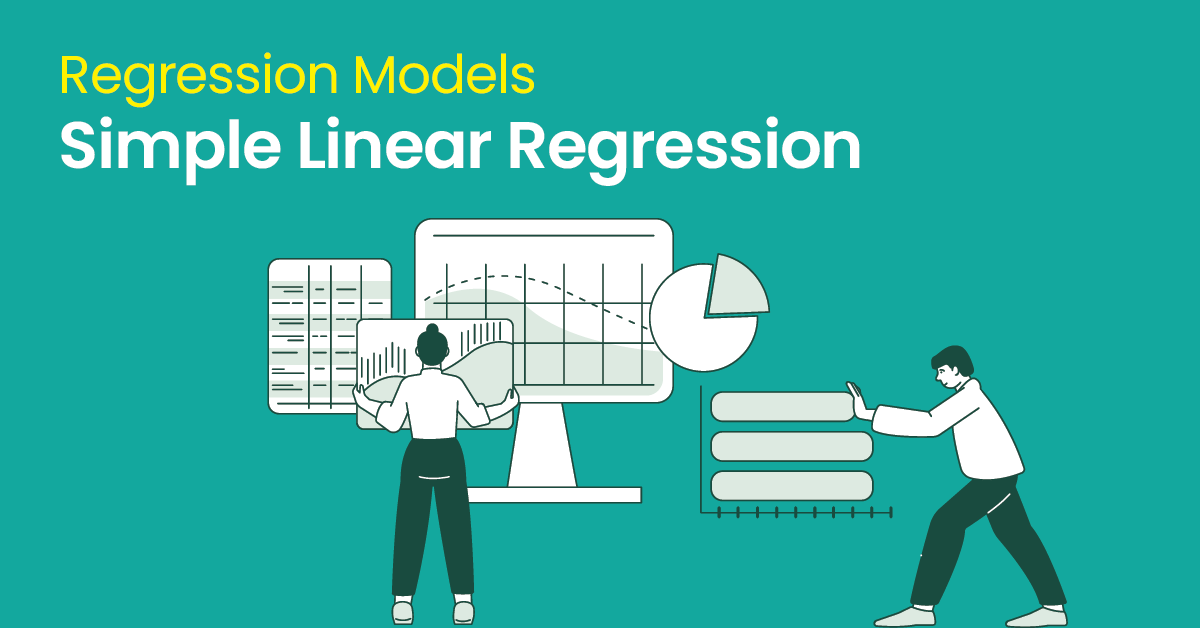### Meet the Author : 360DigiTMG Team

Bharani Kumar Depuru is a well known IT personality from Hyderabad. He is the Founder and Director of Innodatatics Pvt Ltd and 360DigiTMG. Bharani Kumar is an IIT and ISB alumni with more than 17 years of experience, he held prominent positions in the IT elites like HSBC, ITC Infotech, Infosys, and Deloitte. He is a prevalent IT consultant specializing in Industrial Revolution 4.0 implementation, Data Analytics practice setup, Artificial Intelligence, Big Data Analytics, Industrial IoT, Business Intelligence and Business Management. Bharani Kumar is also the chief trainer at 360DigiTMG with more than Ten years of experience and has been making the IT transition journey easy for his students. 360DigiTMG is at the forefront of delivering quality education, thereby bridging the gap between academia and industry.

## Introduction

Suppose you are given a task to solve a mystery. But instead of a magnifying glass and trench coat, you are armed with data and a curious mind. Your mission is to uncover the hidden connection between two variables, to reveal the story lurking beneath the surface. Welcome to the world of simple linear regression, where data is your clue, and the regression equation is your secret decoder. Welcome to my new blog on one of the most important and widely used Supervised technique i.e., Simple Linear Regression. Supervised learning techniques, a subset of machine learning, are widely used in various real-world applications across different domains. These techniques involve training models on labelled data to make predictions or classifications. Such a technique is Simple Linear Regression, it is a fundamental statistical method, that is used to understand the relationship between two continuous variables. It establishes a linear relationship between a dependent and an independent variable. The goal is to find the best-fitting straight line, also known as the regression line or the least-squares line, that describes the relationship between these two variables. We will dive deep into the concepts in this blog.

## What Simple Linear Regression means?

Simple Linear Regression is a supervised learning technique that is used to model the relationship between two continuous variables. It assumes that there is a linear relationship between a dependent variable Y and an independent variable X. Finding a linear equation that best fits the data is the goal.

The equation for the Simple linear Regression is

Y=aX+b

Where

Y is the dependent variable.

X is the independent variable.

a is the slope of the regression line, representing the change in Y for unit change in X.

b is the intercept, representing the value of Y when X is equal to 0.

The primary objective of simple linear regression is to estimate the values of a and b that minimize the sum of the squared differences between the observed values of Y and the values predicted by the linear equation. This is often referred to as the method of least squares.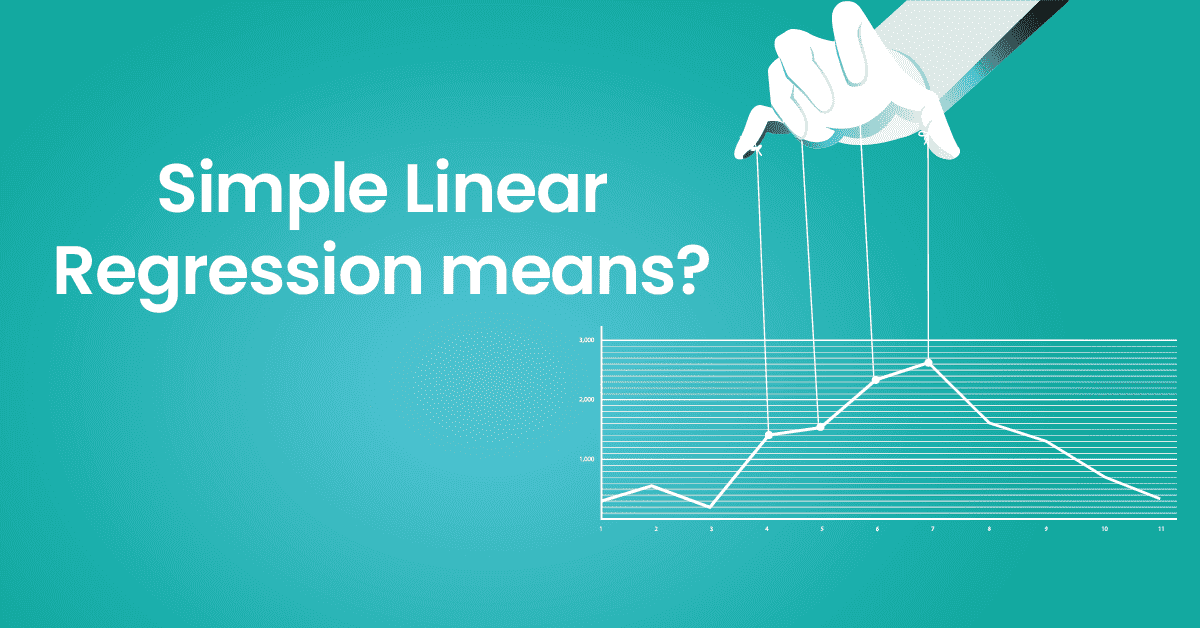If you are using R programming,then to fit the Simple Linear Regression model ‘lm()’ function is used i.e. model <- lm(Y ~ X, data = data) where X is an independent variable and Y is a dependent variable.

If you are using python,then scikit-learn library is used to fit the Simple Linear Regression model.

Now let us check some interesting facts of Simple Linear Regression from google trends:

It has been observed that nearly on an average 60 times per month this word has been searched all over the world.

Let us check the countries where the concept of simple linear regression is followed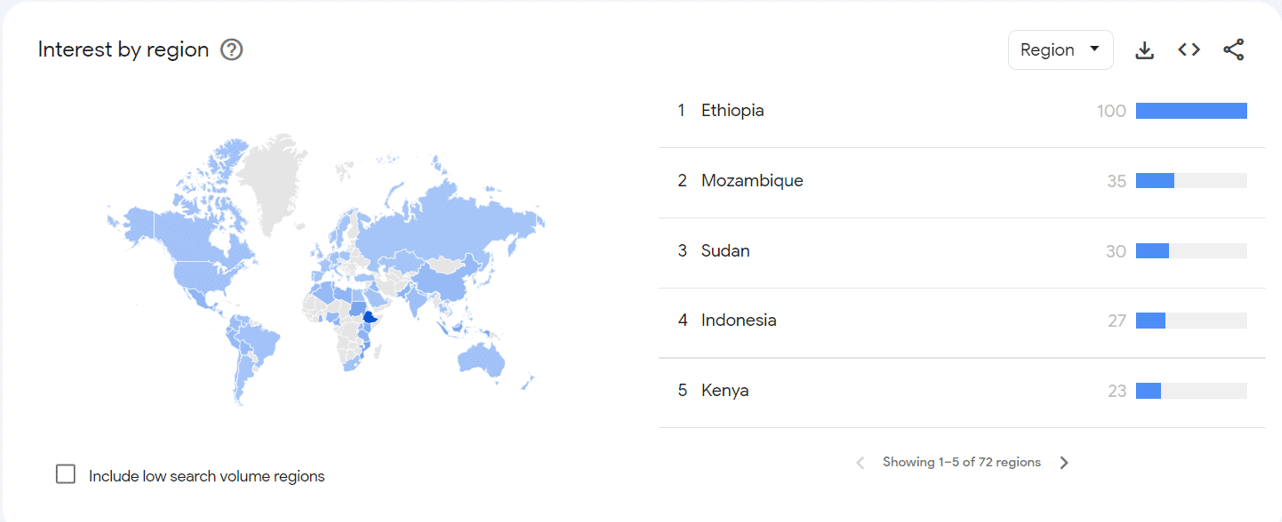As we can see 100 times per month sectors in Ethiopia have searched for Simple linear Regression. From these facts we can see how these supervised learning concepts are searched all over the world.

Earn yourself a promising career in Data Science by enrolling in Data Science Course in Bangalore offered by 360DigiTMG.

## Concepts of Simple Linear Regression

The steps followed in Simple Linear Regression are

• Define the problem: You start with a problem or question that involves two variables: a dependent variable Y and an independent variable X. For example, you might want to understand how the amount of time spent studying X affects a student's exam score Y.
• Collect Data: You gather data on both variables for a sample of observations. In our example, you would collect data on study time X and exam scores Y for multiple students.
• Visualize the Data: Create a scatter plot to visualize the relationship between the two variables. Each data point represents an observation, with X on the horizontal axis and Y on the vertical axis. This step helps you see if there appears to be a linear relationship between X and Y.
• Define the Regression Model: Assume a linear relationship between X and Y, which means you assume that Y can be expressed as a linear function of X, as shown by the equation Y=aX+b. In this equation, a is the slope of the regression line, and b is the intercept.
• Estimate Model Parameters: Use statistical techniques, specifically the method of least squares, to estimate the values of a and b that minimize the sum of squared differences between the observed and predicted values based on the regression equation. These estimated values of a and b represent the best-fit line through the data points.
• Interpret the Results: Once you have estimated a and b, you can interpret the results. The slope a represents the change in Y for a one-unit change in X. In our example, it tells you how much a student's exam score is expected to change for each additional hour spent studying. The intercept b represents the predicted value of Y when X is zero, which may or may not have a meaningful interpretation depending on the context.
• Make Predictions: With the regression equation Y=aX+b,based on the value of X Y can be predicted. For example, you can predict a student's exam score if you know how many hours they studied.
• Evaluate the model: Assess the quality of the regression model. This can be done by calculating various statistics, such as the coefficient of determination (R^2), which measures the proportion of variance in Y that is explained by X. You may also want to examine residual plots to check for any patterns or biases in the model's predictions.
• Conclusions: Based on your analysis, draw conclusions about the relationship between X and Y. For example, you might conclude that there is a statistically significant positive relationship between study time and exam scores.

## OLS method in Simple Linear Regression

OLS stands for Ordinary least squares, and it is a method used in simple linear regression to estimate the parameters of a linear relationship between two variables. It finds the line that best fits the data by minimizing the sum of the squared residuals.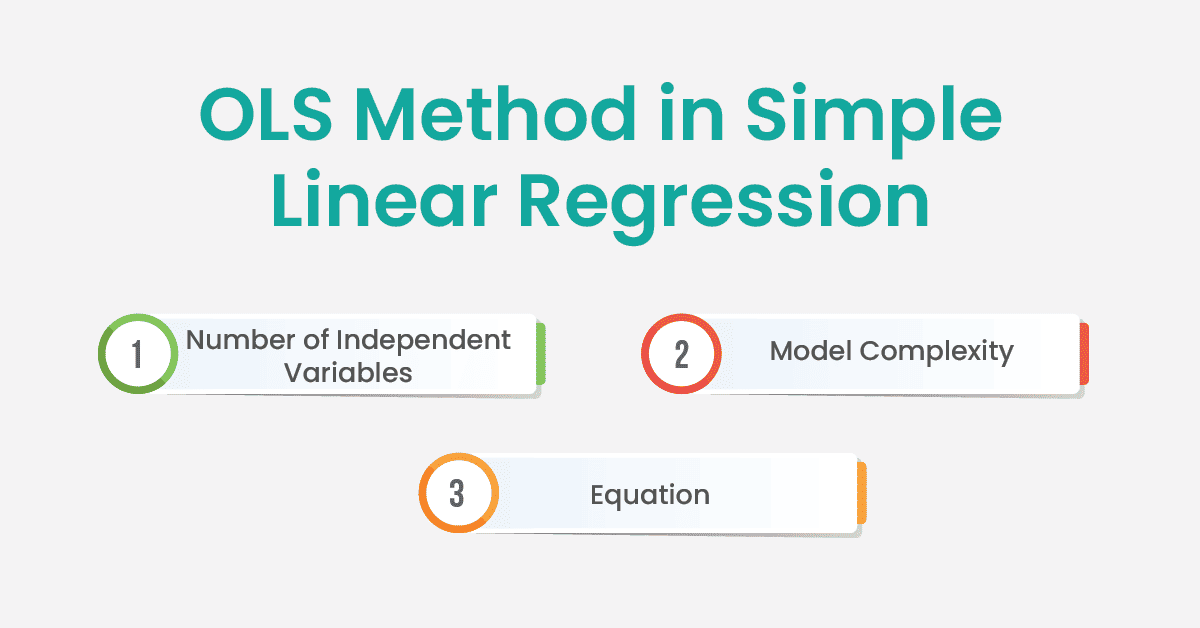## Key Differences between Simple and Multiple Linear Regression.

1.Number of Independent Variables:

• Simple Linear Regression-One independent variable exists here i.e X.
• Multiple Linear Regression- Multiple independent variables present here i.e X1,X2… etc.

2.Model Complexity:

• Simple Linear Regression- one predictor in simpler models.
• Multiple Linear Regression-More complex model that can capture the joint effects of multiple predictors.

3.Equation:

• Simple Linear Regression- One predictor and one coefficient (a) i.e. Y=aX+b.
• Multiple Linear Regression: Multiple predictors and multiple coefficients(a1,a2,a3,..) i.e. Y=a1X1+a2X2+a3X3+b.

Now let us solve one problem using Simple Linear Regression Supervised technique:

We are taking waist circumference and adipose tissue dataset for our analysis. Here we need to predict Adipose Tissue of the body based on Waist Circumference.

Let us see our dataset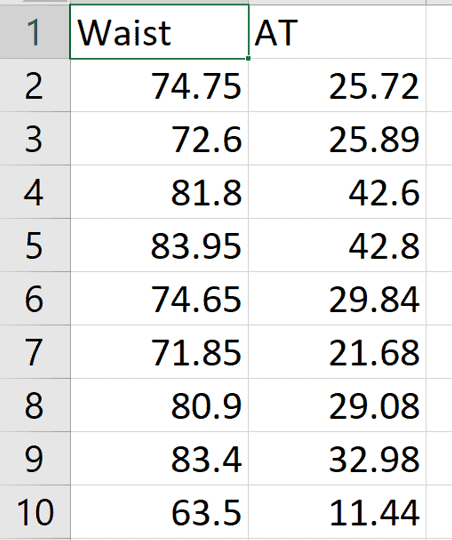Let us do the coding part step by step.

Step 1. Importing necessary libraries and importing the dataset.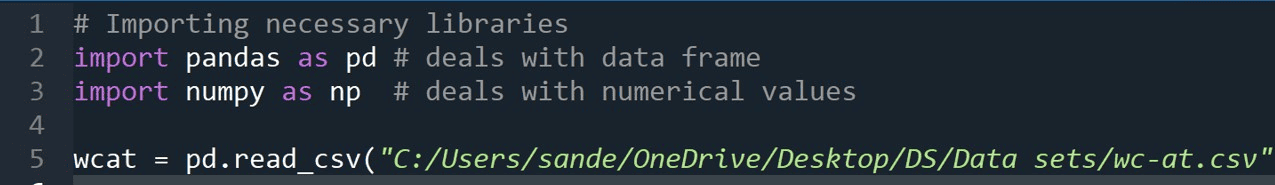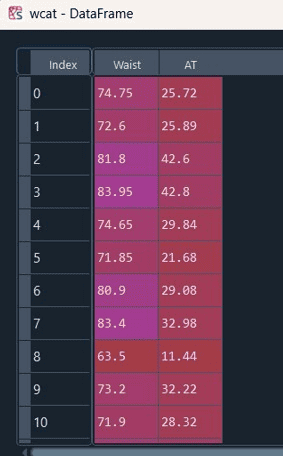This is the Dataframe we have after reading csv file.

Step 2. Checking dimensions of data and getting data description.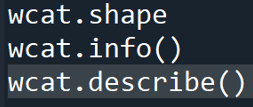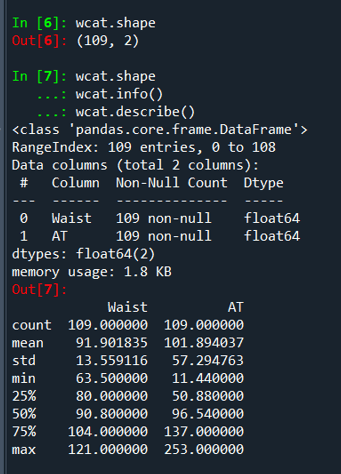As we can see the data dimension is (109,2) that means 109 rows and 2 columns are present and parameters like mean, standard deviation, minimum, maximum etc are given for the dataset.

After preprocessing, we are going to do model building.

Step 3. Importing required libraries for Simple linear Regression and fitting our model.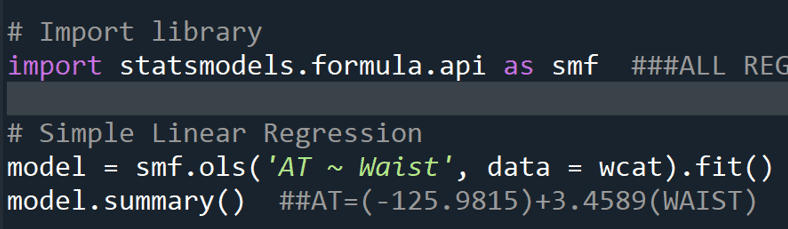Package statsmodel is imported and ols is one of the statistical models used to get coefficients of linear regression equation that creates a relationship between dependent and independent variables.

In our case Waist is the independent variable and AT is the dependent variable and ols method used to minimize the sum of square error between the observed and predicted values.

Now let us check the output and get the summary of the fitted model.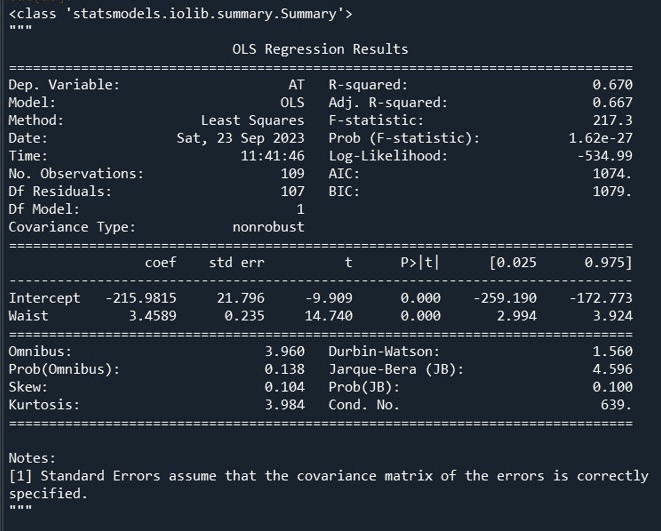The equation we are getting from our model is AT=3.4589*Waist-215.9815.R-Squared and Adj. R-squared determines goodness of fit.67% variability is there in the data and the model is fitting well.

Step 4: Now check the predicted values and find out the error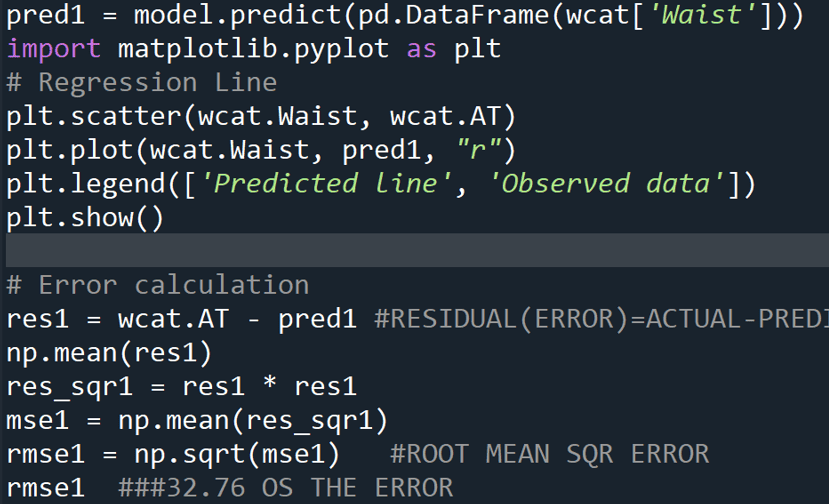The error of 32.76 is coming.

Step 5. Now let us try with some transformation.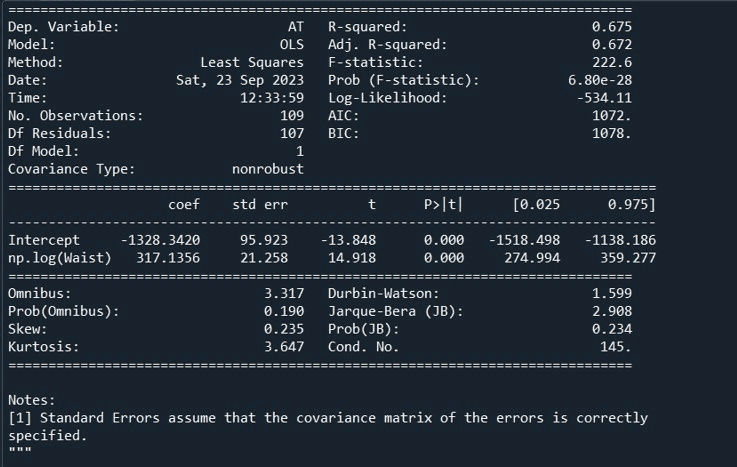We can see R-squared value is 0.675 which has increased from earlier model.

RMSE value is also bit lesser than 1st, model.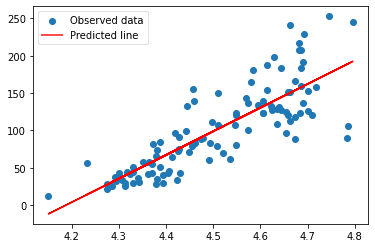We can still see many observed points are not lying on the predicted line.

Become a Data Science Course expert with a single program. Go through 360DigiTMG's Data Science Course Course in Hyderabad. Enroll today!

Step 6:Let us do polynomial transformation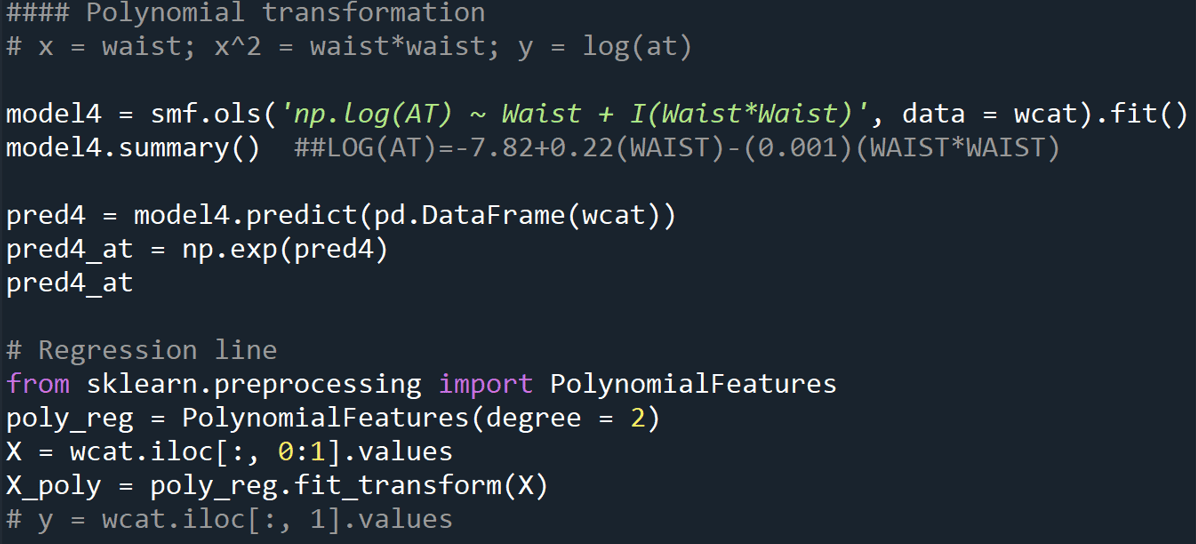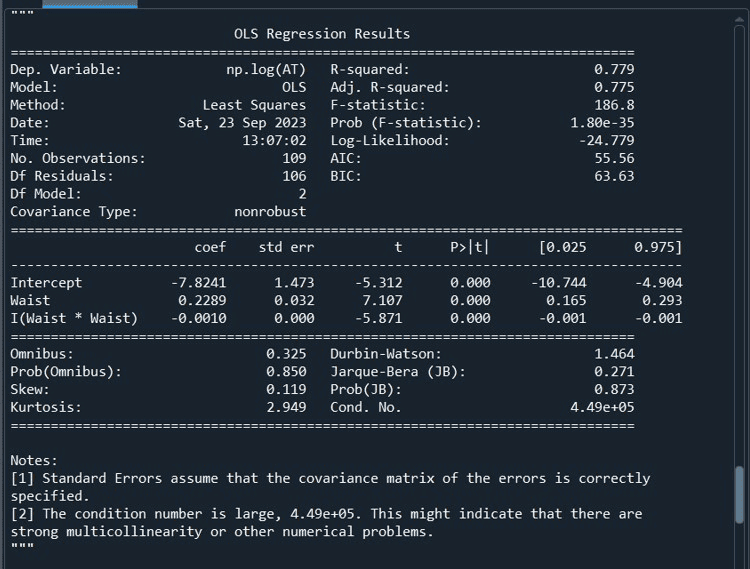This model is good than the previous one, it is because here R-squared value is more. The equation of this model is AT=-7.8241+0.2289*Waist-(0.0010*Waist*Waist)

Data Science, AI and Data Engineering is a promising career option. Enroll in Data Science course in Chennai Program offered by 360DigiTMG to become a successful Career.

Step 7: Let us check the predicted values and calculate the RMSE.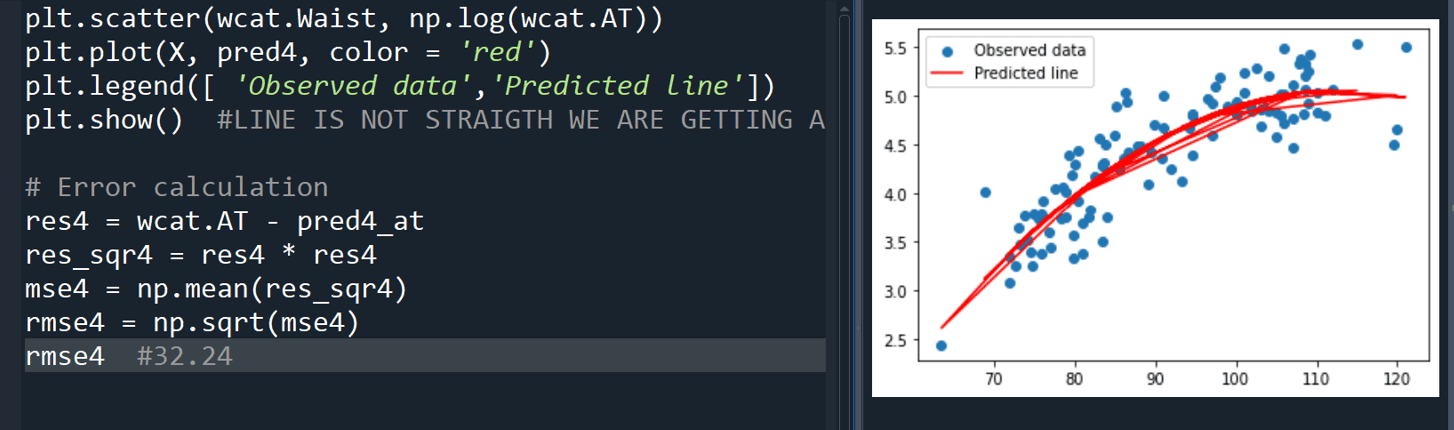As we can see most of the observed values are lying on the line, error is 32.24 which is less than other models we have seen.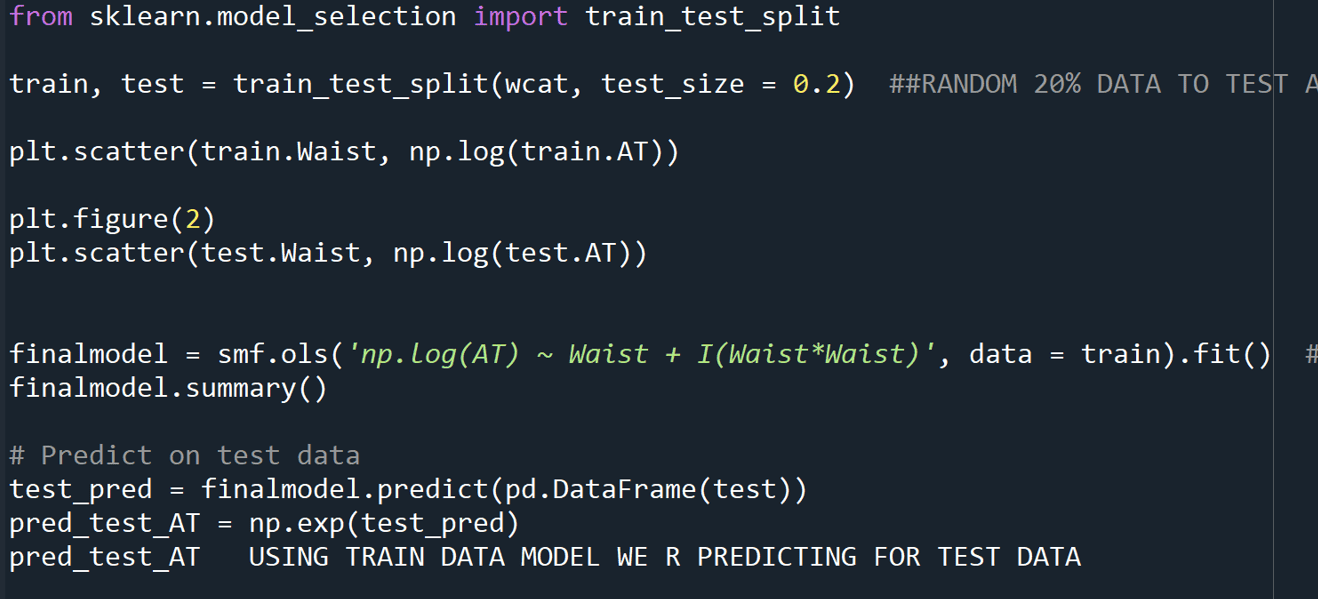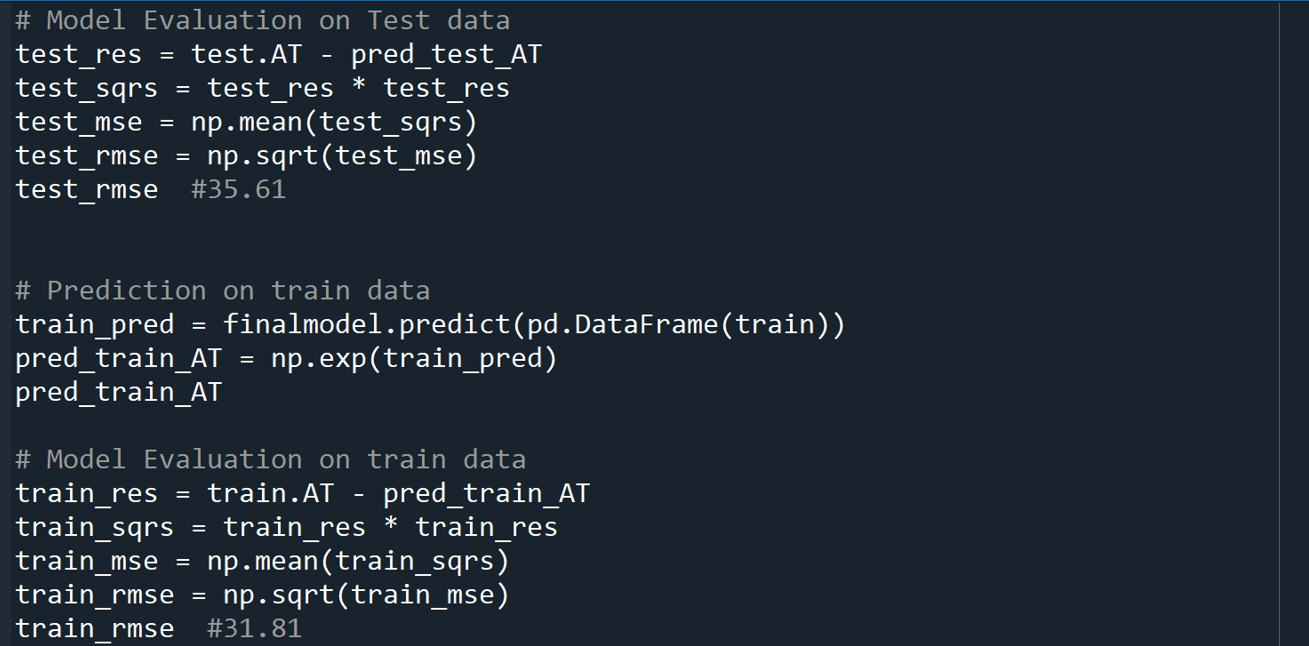Thus, the model giving the least error is having polynomial transformation. We can see the errors for both train and test data are closer to each other and less. Therefore, the model is the right fit model.

## Some Applications of Simple Linear Regression

The following are some of the areas where Simple Linear Regression is used

• Economics and Finance: Simple linear regression is employed in economics to analyse relationships between economic variables, such as the impact of interest rates on consumer spending or the relationship between inflation and unemployment.
• Marketing and Sales: Businesses use simple linear regression for sales forecasting. By analysing historical sales data and factors like advertising expenditure or price changes, companies can make predictions about future sales and adjust their strategies accordingly.
• Medical and Healthcare: Simple linear regression can be applied in healthcare to study the relationship between variables like patient age and medical expenses, drug dosage and treatment outcomes, or patient satisfaction and hospital wait times.
• Sports Analytics: In sports analytics, simple linear regression can be used to analyze player performance metrics (e.g., batting average in baseball or shooting percentage in basketball) and their relationship with factors like training intensity, player fatigue, or coaching strategies.
• Energy and Utilities: Energy companies can use simple linear regression to predict energy consumption based on historical data and weather conditions. This helps in resource planning and optimizing energy distribution.

A lot of areas are still there where linear relationships between variables persist.

## Conclusion

From this blog we learnt what Simple Linear Regression model is, why it is widely used in Data Science, its application in the real world and a code example with Waist circumference Adipose Tissue dataset. In conclusion, simple linear regression offers unique insights into the linear relationship between two variables, making it a valuable tool for understanding and predicting outcomes in various fields. Simple linear regression will continue to be used in the future, alongside more advanced statistical techniques, for various purposes in data analysis and decision-making, its simplicity, interpretability, and historical significance ensure that it will continue to be a valuable and relevant statistical technique in the future. Simple linear regression can be integrated as a feature or as part of a larger predictive modelling process in machine learning and artificial intelligence applications. That is what makes it one of the most popular supervised models in the field of Data Science. Thanks for having patience to read my blog, if you genuinely liked this blog, feel free to give us the feedback in the comment section.

### Data Analyst Courses in Other Locations